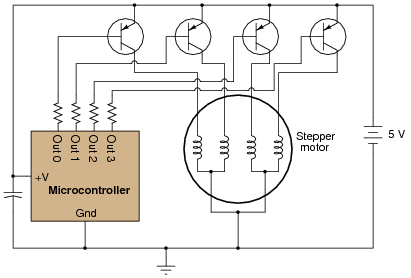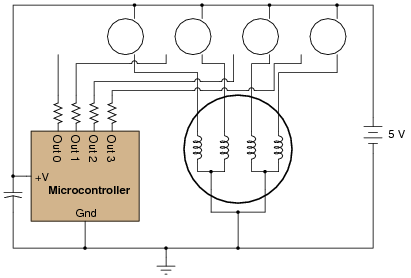# Stepper Motors

## Digital Circuits

• #### Question 1

 Don’t just sit there! Build something!!

Learning to analyze digital circuits requires much study and practice. Typically, students practice by working through lots of sample problems and checking their answers against those provided by the textbook or the instructor. While this is good, there is a much better way.

You will learn much more by actually building and analyzing real circuits, letting your test equipment provide the “answers” instead of a book or another person. For successful circuit-building exercises, follow these steps:

1. Draw the schematic diagram for the digital circuit to be analyzed.
2. Carefully build this circuit on a breadboard or other convenient medium.
3. Check the accuracy of the circuit’s construction, following each wire to each connection point, and verifying these elements one-by-one on the diagram.
4. Analyze the circuit, determining all output logic states for given input conditions.
5. Carefully measure those logic states, to verify the accuracy of your analysis.
6. If there are any errors, carefully check your circuit’s construction against the diagram, then carefully re-analyze the circuit and re-measure.

Always be sure that the power supply voltage levels are within specification for the logic circuits you plan to use. If TTL, the power supply must be a 5-volt regulated supply, adjusted to a value as close to 5.0 volts DC as possible.

One way you can save time and reduce the possibility of error is to begin with a very simple circuit and incrementally add components to increase its complexity after each analysis, rather than building a whole new circuit for each practice problem. Another time-saving technique is to re-use the same components in a variety of different circuit configurations. This way, you won’t have to measure any component’s value more than once.

• #### Question 2

In this circuit, a microcontroller controls the rotation of a special type of motor known as a stepper motor by sequentially activating one transistor at a time (thus, energizing one motor coil at a time). With each step in the sequence, the motor rotates a fixed number of degrees, typically 1.8 degrees per step:Each motor coil draws a relatively heavy current when energized, necessitating transistors to “interpose” between the microcontroller outputs and the motor coils.

Identify what type of logical signal (“high” or “low”) from the output ports of the microcontroller is needed to energize each transistor. Also, show how the power losses and parts count may be reduced by replacing each bipolar junction transistor with a suitable MOSFET in the following diagram:• #### Question 3

Stepper motor coils typically draw a lot of current, requiring the use of power transistors to “buffer” the control circuitry to the motor. A typical stepper motor final drive circuit looks something like this (only one of the four output transistors is shown, for brevity):The diode is installed, of course, to prevent high-voltage surges from destroying the output transistor each time it turns off. However, this causes a different problem: with the free-wheeling diodes in place, the magnetic field formed in each coil takes longer to “decay” when its respective transistor turns off. This delay in time imposes a maximum rotational speed on the stepper motor, because the motor will not move to the next step until the magnetic field(s) from the previous step have dissipated.

What modification may be made to this circuit to allow the transistors to switch faster, driving the stepper motor at a higher rotational speed? Explain in detail why your solution will work.

• #### Question 4

This shift register circuit drives the four coils of a unipolar stepper motor, one at a time, in a rotating pattern that moves at the pace of the clock. The drive transistor circuitry (Q1, Q2, and resistors R2 through R6) are shown only for one of the four coils. The other three shift register outputs have identical drive circuits connected to the respective motor coils:Suppose this stepper motor circuit worked just fine for several years, then suddenly stopped working. Explain where you would take your first few measurements to isolate the problem, and why you would measure there.